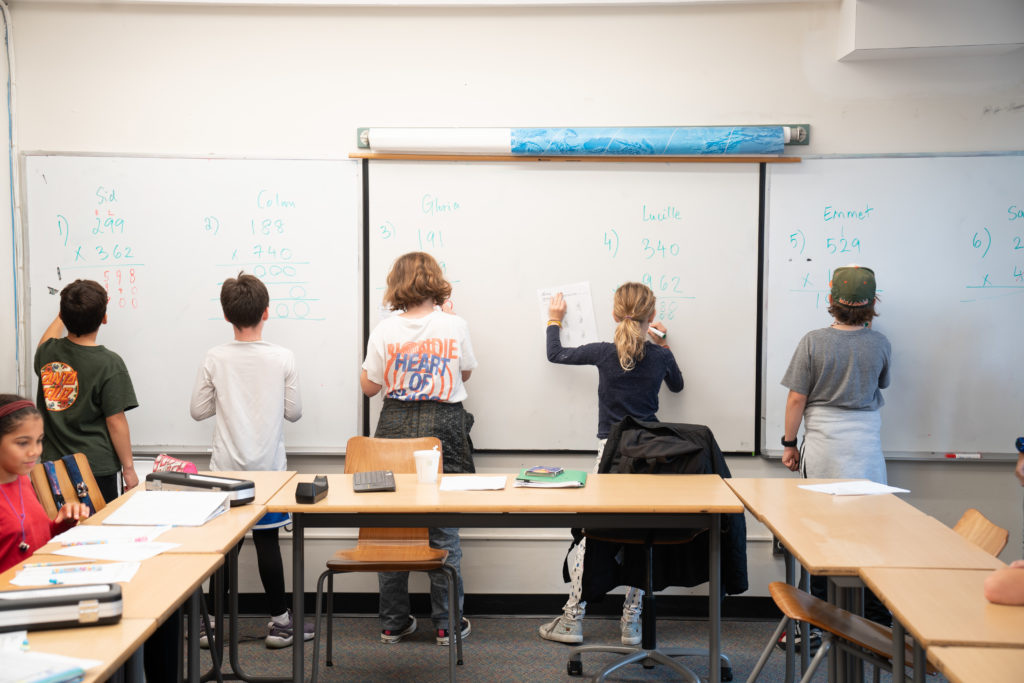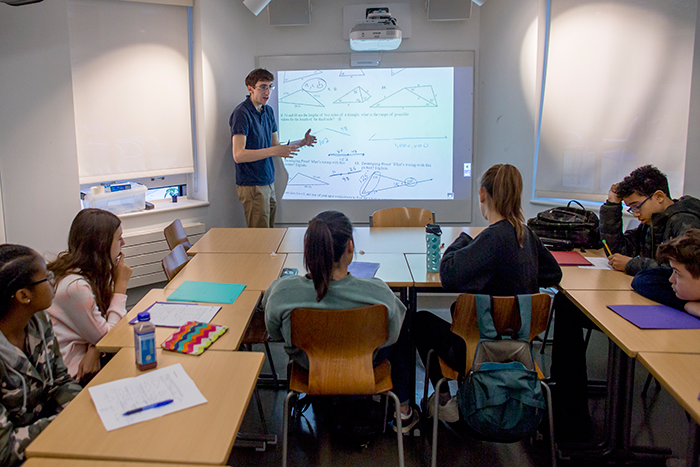# Mathematics: CurriculumThe Mathematics Department at Saint Ann’s is committed to inspiring a sense of joy and confidence in mathematics. We view math as an art form. While exploring the power and beauty of mathematical systems, we aspire to develop thoughtful and precise reasoning in all of our students.

Our teachers pursue deep and compelling questions, challenging our students in their most creative and ambitious moments, while at the same time providing instruction in fundamental principles and algorithms. They lead investigations of number, shape, and pattern, urging students to pose questions, and to communicate their ideas both verbally and in writing. They foster an atmosphere of experimental fervor, encouraging students to make conjectures, to generalize results, and to verify and prove their hypotheses whenever possible. Their freedom to shape the curriculum within guidelines enables them to share their love of mathematics in a way that is most natural for them. Students and teachers develop a comfortable dialogue, and there is also ongoing dialogue among math teachers in which ideas and materials are openly exchanged.

From the Lower School through the High School, classes are formed to accommodate each student’s pacing needs and manner of engagement in the learning process. We strive to make each classroom a vibrant mathematical community where students are able to work together to pursue common goals. The math classes are formed at the end of each school year and are reevaluated and adjusted throughout the subsequent year to ensure that the needs of no individual are compromised.

In the Saint Ann’s Mathematics Department, informal investigations of number, shape, and pattern precede formal manipulations and codification. In Lower School, we explore the workings of base-ten arithmetic and learn to compute using the four operations. We investigate ancient number systems and encourage our students to create their own number systems. We give them the opportunity to play with polygons, tangrams and pentominoes, to develop an intuitive feeling for shape and space. Throughout the Lower School, a math enrichment program introduces mathematical games, puzzles, and construction projects. Students are provided with the opportunity to follow through with these activities throughout the week during free time. We create a body of mathematical experience in a setting that is fun and exciting, and encourage our students to become authors of their own mathematics.

In the Lower Middle School of the fourth and fifth grades, we begin a more formal investigation of base-ten arithmetic. The students’ understanding of the relationship between the four operations and the meaning of a fraction or a mixed number is developed through exploration of the number line, the ruler, fraction bars, and pie charts. The four operations are applied to whole numbers and fractions in problem solving. Counting and strategy games support the student’s awareness and interest in number patterns. Throughout the Lower Middle School, students are encouraged to make observations and form generalizations (for example, is it true that the sum of an odd number and an even number is always odd?).

In the sixth and seventh grades, we begin a more thorough investigation of the real continuum and apply it to the art of measurement. The concept of base-ten place value is extended to the right of the decimal point and we move to the left of zero on the number line to explore the world of negative numbers. The difference between rational and irrational numbers is explored. We examine the concept of a ratio and scaling with respect to similar figures, percents, maps, or architectural drawings, encouraging all of our students to set up equivalent fractions and to think proportionally. Variables are introduced and students begin to model word problems algebraically. They are asked at this point to begin to express generalizations and verify conjectures in abstract form.

Throughout the entire Middle School, we explore topics in logic, number theory, set theory, algebra, geometry, and modular or other-base arithmetic. Our students intuitively explore the concepts of unknowns and balance long before they are introduced to the algebraic laws of equality. They compose and defend logical and geometric arguments long before they are ever asked to perform a formal proof in Geometry. We also offer electives in Problem Solving, Mathematical Art, The Game of Go, and others to provide even more avenues of mathematics for students to explore. Middle school students may participate in various math competitions including MathCounts, AMC 8, and others; some of our students have advanced to the national level of the MathCounts competition.In eighth grade, students begin a formal course in Algebra. It is the first year of high school level mathematics. In Algebra 1, students develop their ability to model and solve word problems by assigning variables and determining the precise relationship between variable expressions. In the process of graphing the solution sets of linear equations on the Cartesian plane, students gain familiarity with the concepts of slope and intercept. They find simultaneous solutions to systems of equations and apply factoring in order to find the roots of quadratic equations. These investigations promote arithmetic and algebraic fluency.

High school students are required to take four years of high school math (one of which is Algebra 1, usually taken in the 8th grade.) All students take Algebra 2 and Geometry. For these classes, students choose between a class that covers fewer topics for longer periods of time and one which moves more quickly through more topics. In addition to this pacing choice, some years a teacher may offer an alternate approach to the subject for any students who are interested (examples have included “Geometry: History and Explorations of Formal Systems” and “Algebra 2: Functions and Abstract Algebra”). Each of these alternate courses takes the place of the corresponding required class, covering the core topics by way of the teacher’s individual approach.

In Geometry, students deepen their appreciation for spatial experience by practicing conjecture and proof as modes of mathematical discourse. Students develop their ability to construct and manipulate configurations of points, lines, circles, and planes in two and three dimensions. While exploring concepts like congruence, similarity, symmetry, and incidence, students organize their observations and generate plausible hypotheses. As they test and critique each other’s theories, they grapple with composing carefully worded definitions and well-crafted proofs. Algebraic tools acquired in previous years help students untangle geometrical relationships and solve measurement problems, while discussions about transformations pave the way for later work with functions.

In Algebra 2, students solve equations, graph relations on the Cartesian plane, and study properties of functions. They use algebraic tools to explore theorems of geometry involving similar figures, right triangles, and properties of a circle. They study conic sections and higher degree polynomials. They derive the quadratic formula and analyze the roots of second-degree equations. This exploration leads to the discovery of complex numbers, the complex plane, and a formulation of the Fundamental Theorem of Algebra. They explore exponential and logarithmic functions. The expansion of the binomial leads to a generalization of the binomial theorem and its application to problems involving counting and probability.

All students also take a weekly Computational Fluency class in the ninth grade. The goal of Computational Fluency is to expose students to the content and format of questions that arise in the SAT and ACT standardized tests. Students are given brief reviews of each topic these tests cover and are then given time to practice solving problems of varying difficulty.

In addition to the required courses, high school students may choose from a variety of electives such as: Trigonometry and Analysis, Calculus 1, Calculus 2, Probability and Statistics, Advanced Problem Solving, Game Theory, Group Theory, Linear Algebra, Non-Euclidean Geometry, Fractals and Chaos, Independent Math Research, Number Theory, Math of Life, Sports Statistics, Introduction to Logic, and a variety of other one-semester courses offered each year. Students also have the opportunity to participate in the New York Math League and American Mathematics Competition. Many of our high school students have gone on to represent the New York City math team in national competitions. On several occasions Saint Ann’s students have represented the United States in international competitions. The breadth of our curriculum reflects the wide range of experience of our faculty in mathematics, science, and the arts.